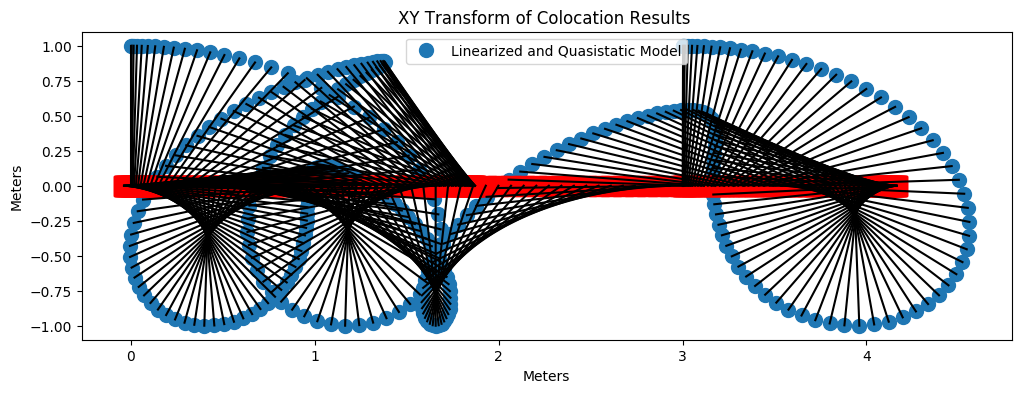Alex Dawson-Elli
Patrick Dills
Kieran Nichols

# Introduction ¶

As Mechanical Engineers, we were inspired to gain new insight into a canonical controls problem though optimization techniques learned in 524. In this report, we develop optimal trajectories for a cart-pole system. The system being modeled consists of a cart and a point mass pendulum. The cart of mass ($m_1$) lays on a frictionless track and can be moved horizontally by control force ($u$). The pendulum with a massless rod (length, $l$) and a bob (mass, $m_2$) is attached to the cart by a frictionless bearing. Because of it's inertia, the force acting on the cart will also cause the pendulum to swing.This class of problem, know as trajectory optimization, has its roots in the Brachistochrone problem posed by Johann Bernoulli in 1697. Brachistochrone problem is to find the path of a ball down a slope which reaches the end in the shortest time. The form of the problem is particularly difficult because it requires optimizing over the set of functions as opposed to optimizing over a variable. To solve in closed form, this required the development of calculus of variation, and is impractical for all but the simplest problems. Modern computers have made the solution of more complex problems trajectory optimization problems possible with applications ranging from computing the paths of rockets to walking robots.

The control problem to be solved may be stated as follows: generate a control signal ($u$) that when applied to the system brings it from an initial configuration (or state) $x_0$ to a desired configuration $x_f$, in the prescribed amount of time. There are infinitely many ways to accomplish this task, but not every trajectory is desirable. For reasons such as reducing component wear, reducing power consumption and increasing smoothness of the path, the heuristics such as minimizing total energy and minimizing sum of the squared force have been used [citation]. In this investigation, we will minimize the square of control force ($u^2$)

In this investigation, we first present a mathematical derivation of the cart-pole dynamics, and standard form of our models. In the Exploration of Modeling Assumptions section we develop 3 models of the same system each of which makes different simplifying assumptions. The assumptions make the problem easier to solve - in the first case this is even enough to change the problem type - at the expense of model fidelity. In the results and discussion section we use three test problems to explore where assumptions are valid and where they break down.

In the final section we investigate the ability of the full dynamics model to swing up the pendulum (from non- inverted to inverted) while avoiding obstacles using either explicit (hard) constraints or regularization (soft constraints). We then develop a model of a double pendulum (cart, two rods, and two bobs) and solve swing up for that system.

citations:

Kelly, Matthew. "An Introduction to Trajectory Optimization: How to Do Your Own Direct Collocation." SIAM Review 59.4 (2017): 849-904.

300 Years of Optimal Control: From The Brachystochrone to the Maximum Principle, Hector J. Sussmann and Jan C. Willems. IEEE Control Systems, 1997.

Posa, Michael, and Russ Tedrake. "Direct trajectory optimization of rigid body dynamical systems through contact." Algorithmic Foundations of Robotics X. Springer, Berlin, Heidelberg, 2013. 527-542

# Mathematical modeling ¶

Cartesian coordinates of the cart and pole will be \begin{equation} \begin{bmatrix} q_1 \\ 0 \end{bmatrix} \qquad \text{and} \qquad \begin{bmatrix} q_1 + l \sin(q_2) \\ l \cos(q_2)] \end{bmatrix} \end{equation} where ($q_1$) is the horizontal position of the cart and ($q_2$) is the angle of the pendulum.

The state, $x$ and state derivative, $\dot{x}$ will give the dynamics of the system in first-order form and represents the minimal generalized coordinates and its derivatives \begin{equation} x = \begin{bmatrix} q_1 \\ q_2 \\ \dot{q_1} \\ \dot{q_2} \end{bmatrix} \qquad \text{and} \qquad \dot{x} = f ( x , u ) = \begin{bmatrix} \dot{q_1} \\ \dot{q_2} \\ \ddot{q_1} \\ \ddot{q_2} \end{bmatrix} \end{equation}

Using Lagrangian method,
$L = T - V$ Where T is the kinetic energy and V the potential energy of the system. L summarizes the system dynamics.

\begin{align} & T = T_{cart} + T_{pole} \\ &T_{cart} = \frac{1}{2} m_1\dot{ q_1}^2 \\ &T_{pole} = \frac{1}{2} m_2 [\dot{(q_1 + l \sin(q_2)}^2 +\dot{(l\cos(q_2)}^2] \\\\ & V = V_{cart} + V_{pole} \\ &V = 0 + m_2 g l \cos(q_2) \\ \end{align}

The potential energy of the cart is zero since it is not moving vertically and it has a height of 0.

Thus $L = \frac{1}{2} m_1 q_1^2 + \frac{1}{2} m_2 [\dot{(x + l \sin(q_2)}^2 +\dot{(l\cos(q_2)}^2] - m_2 g l \cos(q_2)$

The generalized coordinates could be defined as \begin{equation} X = \begin{bmatrix} q_1 \\ q_2 \end{bmatrix} \end{equation} To yield the equations of motion for the cart/pendulum system, the Lagrangian equations will: \begin{align} &\frac{d}{dt}(\frac{\partial L}{\partial{\dot{q_1}}}) - \frac{\partial L}{\partial{\dot{q_1}}} = u \\ &\frac{d}{dt}(\frac{\partial L}{\partial{\dot{q_2}}}) - \frac{\partial L}{\partial{\dot{q_2}}} = 0 \end{align}

Solving for the partial and time derivatives will yield \begin{align} &(m_1 + m_2) \ddot{q_1} + m_1 l \ddot{q_2} \cos(q_2) - m_2 l \dot{q_2}^2 \sin(q_2) = u \\ &m_2 l \ddot{q_1} \cos(q_2) + m_2 l^2 \ddot{q_2} - m_2 g l \sin(q_2) = 0 \end{align} The detailed derivations of the derivatives can be found on this <a href=\"http://www.aoengr.com/Dynamics/PendulumOnCart.pdf\">webisite.</a>

Rearranging the above equations and solving for the $\ddot{q_1}$ and $\ddot{q_2}$ terms respectively, \begin{align} &\ddot{q_1} = \frac{ l m_2 \sin(q_2) \dot{q_2}^2 + u + m_{2} g \cos(q_{2}) sin(q_2)} {m_1 + m_2\sin^2(q_2)} \\ &\ddot{q_2} = \frac{ l m_2 \cos(q_2) \sin(q_2^2) \dot{q_2}^2 + u \cos(q_2) + (m_1 + m_2) g \sin(q_2)} {l (m_1 + m_2\sin^2(q_2))} \end{align}

The objective function will be the continuous integral of the actuator effort (control force) squared: \begin{equation} \min_{u(t)} \int_{t_{initial}}^{t_{final}} u^2 ( t ) d t \end{equation}

The above objective function is a problem with vector functions as decision variables subject to differential equations as constraints. A Transcription process will be used in this project to create a constrained parameter optimization with real numbers as the decision variables that will contain algebraic equations. The continuous time and differential equations will be converted to discrete sets of real numbers by direct collocation where time will be broken into a number of grid points (size N).

\begin{align} % & k \in \{1,\dots,N\}\\ &t_k = {t_0, \dots, t_N}\\ & x_k = x(t_k)\\ & \dot{x_k} = \dot{x}(t_k)\\ & u_k = u(t_k)\\ & h_k = t_{n+1} - t_n \end{align}

To estimate future state and state derivatives ($x_k$ and $\dot{x_k}$), a trapezoidal method will be used where

\begin{align} &\int_{t_{initial}}^{t_{final}} x ( t ) dt \approx \sum_{k = 0}^{N-1} \frac{h_k}{2} (\dot{x_k} + \dot{x_{k+1}}) \end{align}

Additionally, state path constraints were included to ensure that the states were close to the expected trajectory. \begin{align} & {x}_{min} <= {x}_{k} <= {x}_{max}\\ \end{align}

With the direct collocation, the objective function will be the sum of the control force from initial to final step. \begin{equation} \min_{u(t)} \int_{t_{initial}}^{t_{final}} u^2 ( t ) dt \approx \min_{u(k)} \sum_{k = 0}^{N-1} u_k^2 \end{equation}

Restating this optimization problem in discrete standard form will yield \begin{align} \underset{u_k \in {R^2}}{\text{minimize}}\qquad& \sum_{k=1}^{N} u_k^2 \\ \text{subject to:}\qquad & {x_{1}} - {x}_{initial} = 0 && \\ & {\dot{x_{k}}} - {\dot{x}}_{final} = 0 && \\ & {x}_{k} - {x}_{max} <= 0 && \forall k \in \{1,\dots,N\}\\ & -{x}_{k} + {x}_{min} <= 0 && \forall k \in \{1,\dots,N\}\\ & {x}_{k+1} - {x}_{k} - 0.5 h ({\dot x}_{k} +{\dot x}_{k+1}) = 0 && \forall k \in \{1,\dots,N-1\}\\ % & \mathbf{\dot{x}}_{k+1} - \mathbf{\dot{x}}_{k} - % = 0 &&\forall k \in \{1,\dots,k-1\}\\ \end{align}

# Exploration of Modeling Assumptions ¶

### Small Angle Assumption and quazi-static assumptions ¶

This first section is the simplist of the models with linear dynamic constraints to solve the swing up problem. The assumptions include the small angle approximation and quazi-static conditions.

The Small Angle Assumption: $$cos(q_2) \approx 1$$ $$sin(q_2) \approx q_2$$

This simplification removes the trigonometric functions from the dynamic constraints and is usually considered valid for angles of less than 10 degrees about the angle of linearization.

The quazi-static term $$\dot q_2^2 \approx 0$$

The pendulum is assumed to be moving with a small velocity so when that small velocity is sqared, the resulting value is approximately $0$.

The simplified and linear dynamic constraints for the acceleration terms of the cart and pendulum for the optimization are:

\begin{align} & \ddot q_1 = \frac{lm_2sin(q_2)\dot q_2^2 + u + m_2gcos(q_2)sin(q_2)}{m_1 + m_2(1-cos^2(q_2))} \approx \frac{u + m_2gq_2}{m_1} \\ & \ddot q_2 = - \frac{lm_2cos(q_2)sin(q_2)\dot q_2^2 + ucos(q_2) + (m_1+m_2)gsin(q_2)}{lm_1 + lm_2(1-cos^2(q_2))} \approx - \frac{u + (m_1+m_2)gq_2}{lm_1} \end{align}

### Standard Form¶

\begin{aligned} \underset{\mathbf{x},\mathbf{\dot x},\mathbf{u}_i \in \mathbb{R^2}}{\text{minimize}}\qquad& \sum_{i=1}^{n}u_i^2\\ \text{subject to:}\qquad & {x_{1}} - {x}_{initial} = 0 \\ & {\dot{x_{k}}} - {\dot{x}}_{final} = 0 && \\ & {x}_{k} - {x}_{max} <= 0 && \forall k \in \{1,\dots,N\}\\ & -{x}_{k} + {x}_{min} <= 0 && \forall k \in \{1,\dots,N\}\\ & \ddot q_{1k} - \frac{u_k + m_2gq_{2k}}{m_1} = 0 && \forall k \in \{1,\dots,N\}\\ & \ddot q_{2k} + \frac{u_k + (m_1+m_2)gq_{2k}}{lm_1} = 0 && \forall k \in \{1,\dots,N\}\\ & {x}_{k+1} - {x}_{k} - 0.5 h ({\dot x}_{k} +{\dot x}_{k+1}) = 0 && \forall k \in \{1,\dots,N-1\}\\ & \text{where:}\\ & \begin{equation} x = \begin{bmatrix} q_1 \\ q_2 \\ \dot{q_1} \\ \dot{q_2} \end{bmatrix} \qquad \text{and} \qquad \dot{x} = f ( x , u ) = \begin{bmatrix} \dot{q_1} \\ \dot{q_2} \\ \ddot{q_1} \\ \ddot{q_2} \end{bmatrix} \end{equation} \end{aligned}

All of the constraints are linear. However, the quadratic solver Gurobi will be used since the objective function is quadratic.

In :
using JuMP, Gurobi
"""
linearize the dynamics of the cart pull mechanism through the small angle assumption -> first term
in taylor series expansion for sin(q) and cos(q) and quazi-static assumption

inputs:
x0 = [q₁ q₂ q₁dot q₂dot]'      #initial system state
xf = [q₁ q₂ q₁dot q₂dot]'      #final system state
tf = [sec]                     #time to get to final state
h =  [sec]                     #time between collocation points

outputs:
path = [4xk]                   #state matrix that specifies path
"""
function SolvLinearizedCartPole(x0, xf, tf, h , q2const = false, itype = "Trap")

#define model
m = Model(solver = GurobiSolver(OutputFlag=0))
T = 0:h:tf                   #time vector
K = length(T)                 #number of collocation points
@variable(m,    x[1:4,1:K])   #system state at discrete times
@variable(m, xdot[1:4,1:K])   #derivative of system state at discrete times
@variable(m,    u[1:1,1:K])   #control input to system along q1

#boundry constraints - initial and final state
@constraint(m, x[:,1] .== x0)
@constraint(m, x[:,K] .== xf)

#path constraints
#q₂ limit
if q2const
for k in 1:K
@constraint(m, x[2,k] >= xf[2,1] - pi/4)
@constraint(m, x[2,k] <= xf[2,1] + pi/4)
end
end

#q₁ limits
#     for k in 1:K
#         @constraint(m, x[1,k] >= -10)
#         @constraint(m, x[1,k] <=  10)
#     end

#dynamics (path) constraints, integral form
#model constants
l = 1         #length of arm
m1 = 3        #mass of cart
m2 = 1        #mass of pendulum point mass
g = 9.81
linAngle = 0 #the q2 angle we linearize the system about

#setup the dynamics constraints linearized about q1 = 0
if linAngle == 0
for k in 1:K
#notes - linearized system with first term in taylor series, and neglected coreolis acceleration
@constraint(m,xdot[1,k] == x[3,k])
@constraint(m,xdot[2,k] == x[4,k])
@constraint(m,xdot[3,k] == u[1,k] + m2*g*1*x[2,k] / m1)
@constraint(m,xdot[4,k] == -u[1,k]  -(m1 + m2)*g*x[2,k] / m1)

end
end

if linAngle == pi
for k in 1:K
#notes - linearized system with first term in taylor series, and neglected coreolis acceleration
@constraint(m,xdot[1,k] == x[3,k])
@constraint(m,xdot[2,k] == x[4,k])
@constraint(m,xdot[3,k] == u[1,k]  + m2*g*1*x[2,k] / m1)
@constraint(m,xdot[4,k] == u[1,k]  + (m1 + m2)*g*x[2,k] / m1)

end
end

if itype == "forwardEuler"
for k in 1:K-1
@constraint(m, x[:,k+1] .== x[:,k] + h*xdot[:,k])
end

elseif itype == "Trap"
for k in 1:K-1
@constraint(m, x[:,k+1] .== x[:,k] + .5*h*(xdot[:,k] + xdot[:,k+1]))
end
end

# minimize 2-norm (THIS IS LEAST-SQUARES)
@objective(m, Min, sum(u.^2) ) #+ sum(x[2,:]).^2))
solve(m)
#print(m)

control = getvalue(u)
xopt = getvalue(x)
xdotopt = getvalue(xdot)

return (control, xopt,xdotopt)
end

Out:
SolvLinearizedCartPole

### Small Angle Assumption ¶

In this section the complexity of dynamic constraints are increased in an attempt to obtain a more accurate solution to the swing up problem. The small angle assumption is still made to simplify the model. However, the effect of centripetal acceleration on the optimization is included.

Small Angle Assumption: $$cos(q_2) \approx 1$$ $$sin(q_2) \approx q_2$$

Centripetal Acceleration: $$\alpha = lm_2\dot q_2^2$$

This simplification removes the trigonometric functions from the dynamic constraints and is usually considered valid for angles of less than 10 degrees about the angle of linearization.

The simplified dynamic constraints for the optimization are: $$\ddot q_1 = \frac{lm_2sin(q_2)\dot q_2^2 + u_k + m_2gcos(q_2)sin(q_2)}{m_1 + m_2(1-cos^2(q_2))} \approx \frac{lm_2q_2\dot q_2^2 + u + m_2gq_2}{m_1}$$ $$\ddot q_2 = - \frac{lm_2cos(q_2)sin(q_2)\dot q_2^2 + u_kcos(q_2) + (m_1+m_2)gsin(q_2)}{lm_1 + lm_2(1-cos^2(q_2))} \approx - \frac{lm_2q_2\dot q_2^2 + u + (m_1+m_2)gq_2}{lm_1}$$

Both sets of constraints are non-linear and are not able to be expressed in a quadratic or second order cone form because of centripetal acceleration terms, $lm_2q_2\dot q_2^2$, in each of the equations. This forces us to approach the optimization problem with a nonlinear solver like "Ipopt".

### Standard Form¶

\begin{aligned} \underset{\mathbf{x},\mathbf{\dot x},\mathbf{u}_i \in \mathbb{R^2}}{\text{minimize}}\qquad& \sum_{i=1}^{n}u_i^2\\ \text{subject to:}\qquad & {x_{1}} - {x}_{initial} = 0 \\ & {\dot{x_{k}}} - {\dot{x}}_{final} = 0 && \\ & {x}_{k} - {x}_{max} <= 0 && \forall k \in \{1,\dots,N\}\\ & -{x}_{k} + {x}_{min} <= 0 && \forall k \in \{1,\dots,N\}\\ & \ddot q_{1k} - \frac{lm_2q_{2k}\dot q_{2k}^2 + u + m_2gq_{2k}}{m_1} = 0 && \forall k \in \{1,\dots,N\}\\ & \ddot q_{2k} + \frac{lm_2q_{2k}\dot q_{2k}^2 + u + (m_1+m_2)gq_{2k}}{lm_1} = 0 && \forall k \in \{1,\dots,N\}\\ & {x}_{k+1} - {x}_{k} - 0.5 h ({\dot x}_{k} +{\dot x}_{k+1}) = 0 && \forall k \in \{1,\dots,N-1\}\\ & \text{where:}\\ & \begin{equation} x = \begin{bmatrix} q_1 \\ q_2 \\ \dot{q_1} \\ \dot{q_2} \end{bmatrix} \qquad \text{and} \qquad \dot{x} = f ( x , u ) = \begin{bmatrix} \dot{q_1} \\ \dot{q_2} \\ \ddot{q_1} \\ \ddot{q_2} \end{bmatrix} \end{equation} \end{aligned}
In :
using JuMP, Ipopt

"""
simplify the dynamics of the cart pull mechanism through the small angle assumption -> first term
in taylor series expansion for sin(q) and cos(q)

inputs:
x0 = [q₁ q₂ q₁dot q₂dot]'      #initial system state
xf = [q₁ q₂ q₁dot q₂dot]'      #final system state
tf = [sec]                     #time to get to final state
h =  [sec]                     #time between collocation points

outputs:
path = [4xk]                   #state matrix that specifies path
"""
function SolvNonStaticCartPole(x0, xf, tf, h ; q2const = false,  itype = "Trap")

#define model
#m = Model(solver = GurobiSolver(OutputFlag=0))
m = Model(solver = IpoptSolver(print_level=0))
T = 0:h:tf                    #time vector
K = length(T)                 #number of collocation points
@variable(m,    x[1:4,1:K])   #system state at discrete times
@variable(m, xdot[1:4,1:K])   #derivative of system state at discrete times
@variable(m,    u[1:1,1:K])   #control input to system along q1

#boundry constraints - initial and final state
@constraint(m, x[:,1] .== x0)
@constraint(m, x[:,K] .== xf)

#path constraints
#q₂ limit
if q2const
for k in 1:K
@constraint(m, x[2,k] >= xf[2,1] - pi/4)
@constraint(m, x[2,k] <= xf[2,1] + pi/4)
end
end

#q₁ limits
for k in 1:K
@constraint(m, x[1,k] >= -4)
@constraint(m, x[1,k] <=  4)
end

#dynamics (path) constraints, integral form
#model constants
l = 1         #length of arm
m1 = 3        #mass of cart
m2 = 1        #mass of pendulum point mass
g = 9.81
linAngle = pi

#setup the dynamics constraints linearized about q1 = 0
if linAngle == 0
for k in 1:K
#notes - linearized system with first term in taylor series
@constraint(m,xdot[1,k] == x[3,k])
@constraint(m,xdot[2,k] == x[4,k])

q1 = x[1,k] ; q2 = x[2,k] ; q1dot = x[3,k] ; q2dot = x[4,k]
@NLconstraint(m,xdot[3,k] == (l*m2*q2*q2dot^2 + u[1,k] + m2*g*1*q2) / m1)
@NLconstraint(m,xdot[4,k] == -(l*m2*1*q2*q2dot^2 + u[1,k]*1 + (m1 + m2)*g*q2) / l*m1)
end
end

if linAngle == pi
for k in 1:K
#notes - linearized system with first term in taylor series
@constraint(m,xdot[1,k] == x[3,k])
@constraint(m,xdot[2,k] == x[4,k])

q1 = x[1,k] ; q2 = x[2,k] ; q1dot = x[3,k] ; q2dot = x[4,k]
@NLconstraint(m,xdot[3,k] == (l*m2*q2*q2dot^2 + u[1,k] + m2*g*1*q2) / m1)
@NLconstraint(m,xdot[4,k] == -(l*m2*1*q2*q2dot^2 - u[1,k]*1 -(m1 + m2)*g*q2) / l*m1)

end
end

if itype == "forwardEuler"
for k in 1:K-1
@constraint(m, x[:,k+1] .== x[:,k] + h*xdot[:,k])
end

elseif itype == "Trap"
for k in 1:K-1
@constraint(m, x[:,k+1] .== x[:,k] + .5*h*(xdot[:,k] + xdot[:,k+1]))
end
end

# minimize 2-norm (THIS IS LEAST-SQUARES)
#@objective(m, Min, .5*h*sum(u[1:end-1].^2 + u[2:end].^2))
@objective(m, Min, sum(u.^2))
solve(m)

control = getvalue(u)
xopt = getvalue(x)
xdotopt = getvalue(xdot)

return (control, xopt, xdotopt)
end

Out:
SolvNonStaticCartPole

### Full Dynamics ¶

The full dynamic model for a single pendulum is used in this section. There are no simplifying assumptions made to simplify the constraints and a nonlinear solver with nonlinear constraints are necessary.

### Standard Form¶

\begin{aligned} \underset{\mathbf{x},\mathbf{\dot x},\mathbf{u}_i \in \mathbb{R^2}}{\text{minimize}}\qquad& \sum_{i=1}^{n}u_i^2\\ \text{subject to:}\qquad & {x_{1}} - {x}_{initial} = 0 \\ & {\dot{x_{k}}} - {\dot{x}}_{final} = 0 && \\ & {x}_{k} - {x}_{max} <= 0 && \forall k \in \{1,\dots,N\}\\ & -{x}_{k} + {x}_{min} <= 0 && \forall k \in \{1,\dots,N\}\\ & \ddot q_{1k} - \frac{lm_2sin(q_{2k})\dot q_{k2}^2 + u_k + m_2gcos(q_{2k})sin(q_{2k})}{m_1 + m_2(1-cos^2(q_{2k}))} = 0 && \forall k \in \{1,\dots,N\}\\ & \ddot q_{2k} + \frac{lm_2cos(q_{2k})sin(q_{2k})\dot q_{2k}^2 + u_kcos(q_{2k}) + (m_1+m_2)gsin(q_{2k})}{lm_1 + lm_2(1-cos^2(q_{2k}))} = 0 && \forall k \in \{1,\dots,N\}\\ & {x}_{k+1} - {x}_{k} - 0.5 h ({\dot x}_{k} +{\dot x}_{k+1}) = 0 && \forall k \in \{1,\dots,N-1\}\\ & \text{where:}\\ & \begin{equation} x = \begin{bmatrix} q_1 \\ q_2 \\ \dot{q_1} \\ \dot{q_2} \end{bmatrix} \qquad \text{and} \qquad \dot{x} = f ( x , u ) = \begin{bmatrix} \dot{q_1} \\ \dot{q_2} \\ \ddot{q_1} \\ \ddot{q_2} \end{bmatrix} \end{equation} \end{aligned}
In :
using JuMP, PyPlot, Ipopt

#define initial and final states, x
x0 = [0 0 0 0]'
xf = [3 pi 0 0]'   #wind up at the same angle, but

"""
solve the cart pole problem with and full dynamics

inputs:
x0 = [q₁ q₂ q₁dot q₂dot]'      #initial system state
xf = [q₁ q₂ q₁dot q₂dot]'      #final system state
tf = [sec]                     #time to get to final state
h =  [sec]                     #time between collocation points

outputs:
path = [4xk]                   #state matrix that specifies path
"""
function SolvFullCartPole(x0, xf, tf, h; q2const = false, xStart="None" , itype="Trap")

#define model
m = Model(solver = IpoptSolver(print_level=0))
T = 0:h:tf                   #time vector
K = length(T)                 #number of collocation points
@variable(m, x[1:4,1:K])   #system state at discrete times
@variable(m, xdot[1:4,1:K])   #derivative of system state at discrete times
@variable(m,    u[1:1,1:K])   #control input to system along q1
if xStart != "None"  setvalue(x[:,:] , xStart) end

#boundry constraints - initial and final state
@constraint(m, x[:,1] .== x0)
@constraint(m, x[:,K] .== xf)

#path constraints
if q2const
for k in 1:K
@constraint(m, x[2,k] >= xf[2,1] - pi/4)
@constraint(m, x[2,k] <= xf[2,1] + pi/4)
end
end

for k in 1:K
@constraint(m, x[1,k] >= -10)
@constraint(m, x[1,k] <=  10)
end

#model constants
l = 1         #length of arm
m1 = 3        #mass of cart
m2 = 1        #mass of pendulum point mass
g = 9.81

#setup the full dynamics constraints
for k in 1:K
@constraint(m,xdot[1,k] == x[3,k])
@constraint(m,xdot[2,k] == x[4,k])
q1 = x[1,k] ; q2 = x[2,k] ; q1dot = x[3,k] ; q2dot = x[4,k]
@NLconstraint(m,xdot[3,k] ==  (l*m2*sin(q2)*q2dot^2 + u[1,k] + m2*g*cos(q2)*sin(q2)) /
(m1 + m2*(1-cos(q2)^2)))
@NLconstraint(m,xdot[4,k] == -(l*m2*cos(q2)*sin(q2)*q2dot^2 + u[1,k]*cos(q2) + (m1 + m2)*g*sin(q2))/
(l*m1 + l*m2*(1-cos(q2)^2)))
end

if itype == "forwardEuler"
for k in 1:K-1
@constraint(m, x[:,k+1] .== x[:,k] + h*xdot[:,k])
end

elseif itype == "Trap"
for k in 1:K-1
@constraint(m, x[:,k+1] .== x[:,k] + .5*h*(xdot[:,k] + xdot[:,k+1]))
end
end

# minimize 2-norm (THIS IS LEAST-SQUARES)
#@objective(m, Min, .5*h*sum(u[1:end-1].^2 + u[2:end].^2))
@objective(m, Min, sum(u.^2) ) # + 10000*sum(x[2,:] - pi).^2)
solve(m)
#print(m)

control = getvalue(u)
xopt = getvalue(x)
xdotopt = getvalue(xdot)

return (control, xopt, xdotopt)
end

Out:
SolvFullCartPole
In :
using PyPlot

# Plotting the output of the Optimization in q coords and effort
function plotq2(x,h,tf)
#figure(figsize=(12,4))
title("q_2 trajectory")
xlabel("time[sec]")
t = 0:h:tf
plot( t, x[2,:], markersize = 2)
end

function plotq1(x,h,tf)
title("q_1 trajectory")
xlabel("time[sec]")
ylabel("Distance [m]")
t = 0:h:tf
plot( t, x[1,:], markersize = 2)
end

function plotu(u,h,tf)
title("Optimization Output Effort")
xlabel("time[sec]")
ylabel("Force [N]")
plot(u[1,:])
end

# plotting the cart and pendulum position at each collocation point
function plotTipTraj(x)
#Transform to x and y coordinates of the mass location
l = 1
K = size(x)
xCordM = zeros(1,K)
yCordM = zeros(1,K)

xCordM = x[1,:] + l*sin.(x[2,:])
yCordM = -l*cos.(x[2,:])

title("trajectory of tip in xy plane ")
xlabel("Meters")
ylabel("Meters")
plot( xCordM, yCordM,".", markersize = 2)
end

# Plotting a stem plot of the cart and pendulum position at each colocation point
function plotXY(x)
l = 1
K = size(x)

# Transform to x and y coordinates of the tip location
xCordM = zeros(1,K)
yCordM = zeros(1,K)
xCordM = x[1,:] + l*sin.(x[2,:])
yCordM = -l*cos.(x[2,:])

# Transform to x and y coordinates of the Cart
xCordC = zeros(1,K)
yCordC = zeros(1,K)
xCordC = x[1,:]'

#figure(figsize=(12,4))
title("XY Transform of Colocation Results ")
xlabel("Meters")
ylabel("Meters")
plot( xCordM, yCordM,".", markersize = 20);
plot( xCordC, yCordC,"rs", markersize = 15);
#legend(["Mass Location","Cart Location"])
for i =1:length(xCordM)
plot( [xCordM[i],xCordC[i]] , [yCordM[i], yCordC[i]],"k");
end
end

function plotContour(xoff,yoff)
xv = linspace(-6,4,1000)
yv = linspace(-1.1,1.1,1000)
function meshgrid{T}(vx::AbstractVector{T}, vy::AbstractVector{T})
m, n = length(vy), length(vx)
vx = reshape(vx, 1, n)
vy = reshape(vy, m, 1)
(repmat(vx, m, 1), repmat(vy, 1, n))
end
(X,Y) = meshgrid(xv,yv);

z = [1/(sqrt(2*pi*sig^2))*exp(-((x-xoff)^2 + (y-yoff)^2)/(2*sig^2)) for y in yv, x in xv]
contour(X, Y, z, zdir="z", 60, offset=0, linewidths=.5 );
end

# Plotting the Ellipse Constraining the motion of the pendulum
function plotEllipse(a,b,c,xoff,yoff)
angle = linspace(0,2*3.14595,360);
r = zeros(1,length(angle));
xE = zeros(1,length(angle));
yE = zeros(1,length(angle));
for i = 1:length(angle);
r[i] = a*b/sqrt(b^2*cos(angle[i])^2 + a^2*sin(angle[i])^2);
xE[i] = r[i]*cos(angle[i]) + xoff;
yE[i] = r[i]*sin(angle[i]) + yoff;
end
plot(xE,yE,"g.--");
end

Out:
plotEllipse (generic function with 1 method)

### Test Case 1) $x_0 = [0, 0, 0, 0]'$ ; $x_f = [3, 0, 0, 0]'$¶

visualization of test case 1 performed on full dynamics model

In this test case, the system is moving about a stable equilibrium point at $q_2 = 0$, meaning that any displacement in $q_2$ results in a restoring torque due to gravity. Because the total time execution is long for the given distance (3meters), average velocity of the cart is kept low, and angular deviation of $q_2$ is small. Also, angular velocity of q2 is kept at a minimum. This leads us to believe that the linearized model, which makes both small angle and quazi-static assumptions, and the intermediate model, which makes small angle assumptions, will produce accurate results for this test case.

In :
#define initial and final states
x0 = [0 0 0 0]'
xf = [3 0 0 0]'
tf = 3 ; h = .01

#evaluate each model
u1,x1,xdot1 =  SolvLinearizedCartPole(x0, xf, tf, h ; q2const = false , itype = "Trap")
u2,x2,xdot2 =  SolvNonStaticCartPole(x0, xf, tf, h ; q2const = false,  itype = "Trap")
u3,x3,xdot3 =  SolvFullCartPole(x0, xf, tf, h;  q2const = false, xStart="None" , itype= "Trap");

Academic license - for non-commercial use only

In :
#plot the resulting tip trajectory
figure(figsize=(12,4))
plotTipTraj(x1)
plotTipTraj(x2)
plotTipTraj(x3)
legend(["small angle + quazi-static","small angle", "Full Dynamics" ])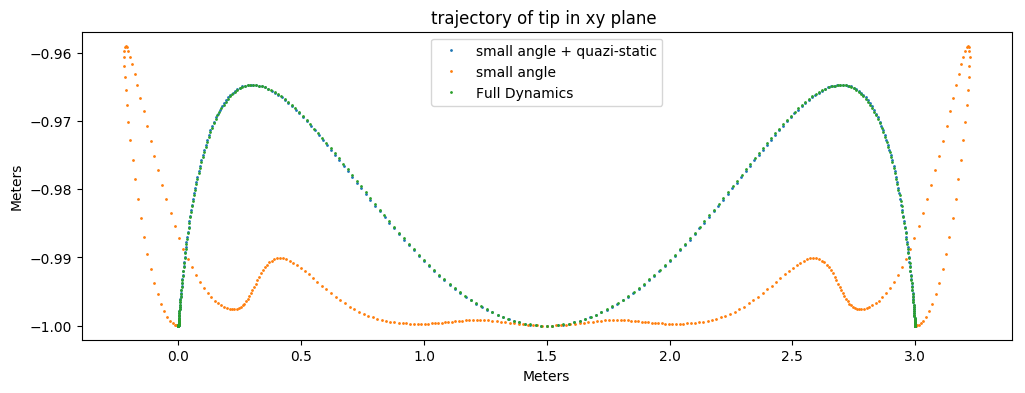Out:
PyObject <matplotlib.legend.Legend object at 0x00000000A9F4BE10>
In :
#plot q1 vs. time
figure(figsize=(12,4))
plotq1(x1,h,tf)
plotq1(x2,h,tf)
plotq1(x3,h,tf)
legend(["small angle + quazi-static","small angle", "Full Dynamics" ])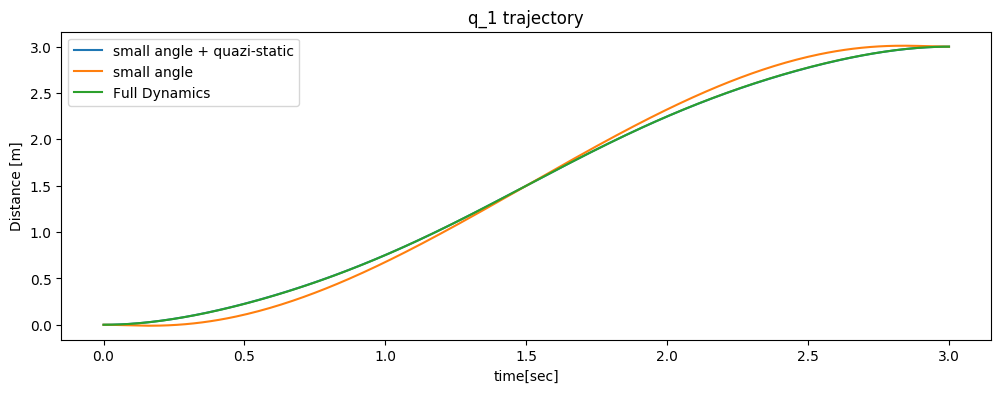Out:
PyObject <matplotlib.legend.Legend object at 0x0000000053BC4DD8>
In :
figure(figsize=(12,4))
plotq2(x1,h,tf)
plotq2(x2,h,tf)
plotq2(x3,h,tf)
legend(["small angle + quazi-static","small angle", "Full Dynamics" ])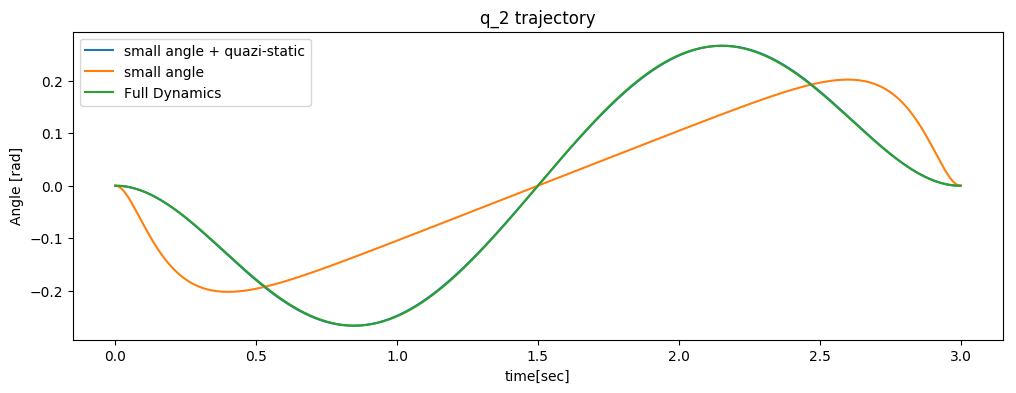Out:
PyObject <matplotlib.legend.Legend object at 0x00000000548853C8>
In :
figure(figsize=(12,4))
plotu(u1)
plotu(u2)
plotu(u3)
legend(["small angle + quazi-static","small angle", "Full Dynamics" ])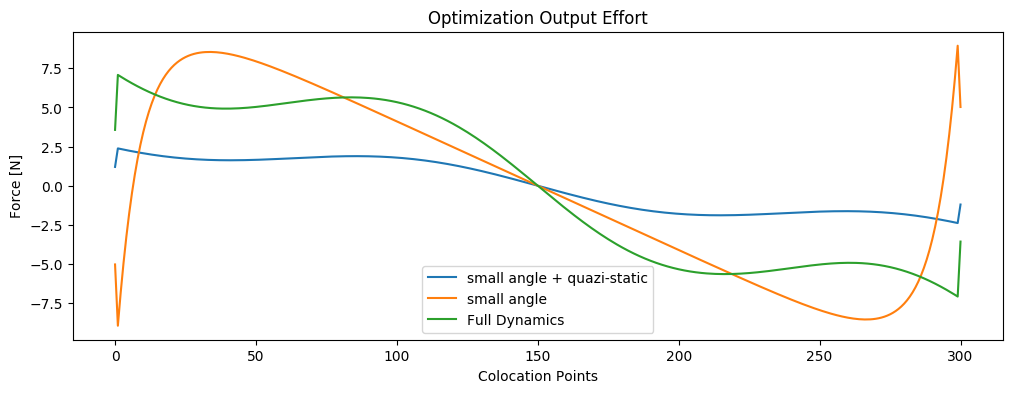Out:
PyObject <matplotlib.legend.Legend object at 0x0000000056681F98>

### Test Case 2) $x_0 = [0, \pi, 0, 0]'$ ; $x_f = [3, \pi, 0, 0]'$¶

visualization of test case 2 performed on full dynamics model

In this test case, the system is moving about a unstable equilibrium point at $q_2 = \pi$, meaning that any displacement in $q_2$ results in a restoring torque due to gravity. Because the total time execution is long for the given distance (3meters), average velocity of the cart kept low, and angular deviation of $q_2$ is small. In addition, the angular velocity of q2 is kept at a minimum. This leads to the prediction that the linearized model, which makes both small angle and quazi-static assumptions, and the intermediate model, with small angle assumptions, should produce accurate results for this test case.

We tested a constrained and unconstrained version of this problem. All three cases were solved in the unconstrained case. However only the full dynamics were able to be solved in the constrained case shown above.

### Unconstrained¶

In :
#define initial and final states
x0 = [0 pi 0 0]'
xf = [3 pi 0 0]'
tf = 3 ; h = .01

#evaluate each model
u1,x1,xdot1 =  SolvLinearizedCartPole(x0, xf, tf, h ; q2const = false , itype = "Trap")
u2,x2,xdot2 =  SolvNonStaticCartPole(x0, xf, tf, h ; q2const = false,  itype = "Trap")
u3,x3,xdot3 =  SolvFullCartPole(x0, xf, tf, h;  q2const = false, xStart="None" , itype= "Trap");

Academic license - for non-commercial use only

In :
#plot q1 vs. time
figure(figsize=(12,4))
plotq1(x1,h,tf)
plotq1(x2,h,tf)
plotq1(x3,h,tf)
legend(["small angle + quazi-static","small angle", "Full Dynamics" ])
figure(figsize=(12,4))
plotq2(x1,h,tf)
plotq2(x2,h,tf)
plotq2(x3,h,tf)
legend(["small angle + quazi-static","small angle", "Full Dynamics" ])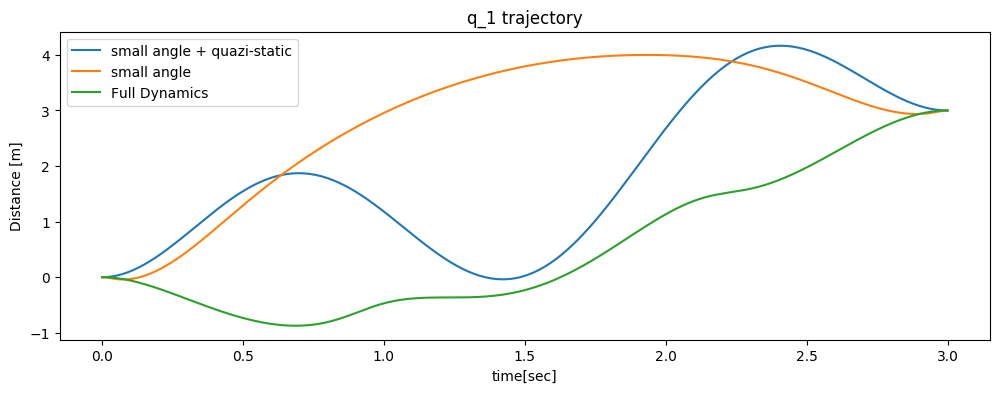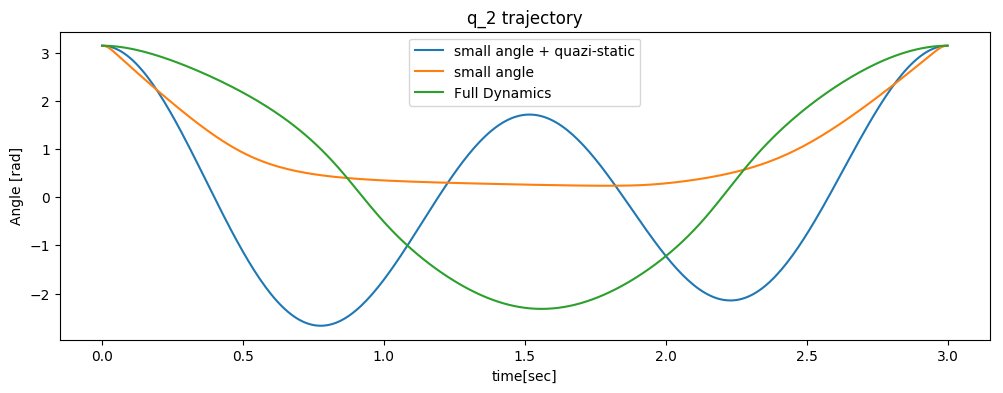Out:
PyObject <matplotlib.legend.Legend object at 0x00000000A62ED080>
In :
figure(figsize=(12,4))
plotXY(x1)
legend(["Linearized and Quasistatic Model"])
figure(figsize=(12,4))
plotXY(x2)
legend(["Linearized Model"])
figure(figsize=(12,4))
plotXY(x3)
legend(["Full Dynamics"])